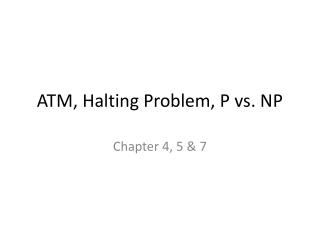# ATM, Halting Problem, P vs. NP - PowerPoint PPT PresentationDownload PresentationATM, Halting Problem, P vs. NP

ATM, Halting Problem, P vs. NPDownload Presentation## ATM, Halting Problem, P vs. NP

- - - - - - - - - - - - - - - - - - - - - - - - - - - E N D - - - - - - - - - - - - - - - - - - - - - - - - - - -
##### Presentation Transcript

1. ATM, Halting Problem, P vs. NP Chapter 4, 5 & 7

2. Russel’s Paradox • http://www.jimloy.com/logic/russell.htm • An Index is a book that lists other books in the library • Index of all biology text books in the library. • Consider the index of all indices, i.e., book that lists other indices. • It would contain itself. • Called a self-referential index (form of recursion). • Consider the index of all non self-referential indices. • The book that lists all indices that are non self-referential. • Call it the non-recursive index • If the non-recursive index lists itself then its recursive and shouldn’t be in the list. • But if it doesn’t list itself, then it is non-recursive and it should be listed as one of the non self-referential books.

3. ATM reduces to HALTTM • Proof by Contradiction • Assume we have a Turing Machine R that decides HALTTM. • In other words, we can design an algorithm that determines of another algorithm (M) will halt on a given input (w).

4. ATM reduces to HALTTM • Proof by Contradiction • Assume we have a Turing Machine R that decides HALTTM. • Then, use R to construct a new machine S that can decide ATM. • We have already proven that is NOT decidable. • So, if we can construct a machine S that can decide ATM based on HALTTM then we must conclude that cannot be decidable.

5. ATM reduces to HALTTM • Proof by Contradiction • Assume we have a Turing Machine R that decides HALTTM. • Then, use R to construct a new machine S that can decide ATM. • We have already proven that ATM is NOT decidable. • So, if we can construct a machine S that can decide ATM based on HALTTM then we must conclude that cannot be decidable.

6. Deeply Understanding ATM • ATM is just a set of strings where part of the string is the encoding of a Turing Machine (M) and the other part is an input string (w). • The string in ATM are the ones were the input w is accepted by the Machine M. • The string in the complement of are the ones the input w is rejected by the Machine M. • A decider for ATM cannot exist because there are absurd Turing Machines infinite loop or output nothing. • Detecting such absurdity is impossible because you are limited by the power of the same machine that allows for such absurdity. • Simulating an absurd Turing Machine with another Turing Machine cannot “undo” it absurdity and allow it to always produce an answer (accept or reject).

7. Chapter 7 Time Complexity

8. Big-O

9. Little-o

10. O(n2)

11. O(n log2n)

12. O(n)

13. Non-determinism

14. The Cost of Non-determinism

15. The Class P

16. Example of a problem in P

17. PATH is polynomial

18. The Class NP

19. Polynomial Verification • Some problems (Hamiltonian Path) cannot be solved in Polynomial Time (P), but… • If we could somehow obtain a solution (ask an oracle), the solution could be verified in P-time.

20. Hamiltonian Path: Decider vs. Verifier • There is very subtle difference: • Hamiltonian Decider • Input: Graph • Output: Hamiltonian Path • Hamiltonian Verifier • Input: Graph, Hamiltonian Path • Output: Accept/Reject

21. Hamiltonian Path Algorithm – Non-deterministic

22. Understand NP in English • Problems where the solutions can be verified in P-Time, but cannot be “discovered” in P-time • Problems where the solutions can be “discovered” P-Time using a Non-deterministic Turing Machine • Note: Every non-deterministic Turing machine can be made deterministic by adding O(kn)